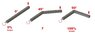# Levers, torque and the (non?) linear relationship

• hlock

#### hlock

Homework Statement
What is the relative force applied to the bar bend to 45 degrees?
Relevant Equations
unknown
I'm unsure what equation to use to achieve this. My solution was to draw a scale model and measure the distance offset from the bar and how relative that was to the same length bar bent to 90 degrees.

My answer was 70.8% assuming a linear relationship between length of lever and force (is that right?). I've made the possibly incorrect assumption that if you double the length of a lever you double the force.

The answer requires a descriptive answer relating angle to force applied, so to me it doesn't appear linear (or it would be 50%?) but what is it? How best describes the relationship?

#### Attachments

•levers.jpg
19 KB · Views: 96
Last edited:
•berkeman
This type of problems should always define the axis of rotation, as well as the direction of the force in relation to that axis.
The schematic shows percentages of torque at one end, but at the same time asks about intensity of applied force.
It seems to me that they should assume the same force is applied for the three cases.